Applied and Computational Mathematics
Volume 5, Issue 2, April 2016, Pages: 56-63

Effect of Grid Step Sizes on Computational Time Using Finite-Difference Method for Seismic Wave Modeling

Olowofela Joseph. A.1, Akinyemi Olukayode. D.1, Ajani Olumide. O.2

1Department of Physics, Federal University of Agriculture (FUNAAB), Abeokuta, Nigeria

2Department of Physics and Solar Energy, Bowen University, Iwo, Nigeria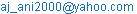(Ajani Olumide. O.)

Olowofela Joseph. A., Akinyemi Olukayode. D., Ajani Olumide. O. Effect of Grid Step Sizes on Computational Time Using Finite-Difference Method for Seismic Wave Modeling. Applied and Computational Mathematics. Vol. 5, No. 2, 2016, pp. 56-63. doi: 10.11648/j.acm.20160502.14

Received: September 5, 2015; Accepted: September 21, 2015; Published: April 15, 2016

Abstract: Finite-difference method, a popular seismic forward modeling method is a technique which allows us to numerically solve partial differential equations like the wave equation solved in this paper. Beyond its use in standard data acquisition, it is a very instructive tool to understand how waves propagate in the earth's subsurface. Since the accuracy obtainable by using the finite difference scheme lies solely on its stability and ability to handle grid dispersion, this is achievable by applying appropriate grid step sizes. The developed finite-difference method was employed to generate snapshots of synthetic seismograms to highlight the effect of grid step sizes on computational time while ensuring numerical stability of the scheme used through accurate discretization of the medium and adopting Perfectly Matched Layer (PML) absorbing boundary conditions. Results shows that for a grid size of 5m x 5m x 5m having 260 x 260 x 100 grid points and time step of 100 - 500, the wavefield propagating is horizontally symmetric. From the results, the importance of grid step sizes on computational time is re-emphasized.

Keywords: Finite Difference Method, Forward Modeling, Synthetic Seismogram, Stability, Wavefield, Perfectly Matched Layer

Contents

1. Introduction

Finite-difference method is a popular seismic forward modeling method. It is a numerical method for solving partial differential equations. It is useful in the computation of seismic displacement 'u' or seismic velocity 'v' at any point in a given geological model when applied to seismic wave equation of motion. The finite-difference method is used to compute the wave fields u(x,y,z,t) or v(x,y,z,t) at a discrete set of closely-spaced grid points (xi, yj, zk,tp), with i,j,k,p = 0,1,2,3,4,5,........, by approximating the derivatives occurring in the equation of motion with finite-difference formula, and recursively solving the resulting difference equation. However, owning to the importance of the need for accuracy of the computed solution, using this method, a reliable way of ensuring this is to use smaller grid time-step sizes while ensuring that the stability condition is satisfied. It should be noted that other methods of improving the accuracy of finite-difference exist, example is the use of higher-order approximations for the derivatives but they usually result in more complicated and cumbersome finite-difference scheme and in an increased computational time (Moczo et al., 2007).

Against this background, this paper examines the effect of various grid step sizes on computational time while employing the finite-difference scheme to model seismic wave propagation in a 2-layered medium using simulation script written in MATLAB.

2. Methodology

The finite-difference method used for spatial discretization of the governing equations used in the modeling work carried out in this research is explained in more details here (Extracted from notes by Moczo et al., 2004). Only fully elastic media and in viscid fluids are modeled with the finite difference method. A velocity - stress finite difference formulation is used and the guiding equations formulated in the following way: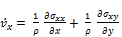(1)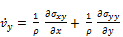(2)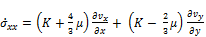(3)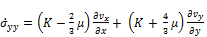(4)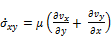(5)

Equations 1 - 5 represent five first order partial differential equations for the five unknowns vx, vy, σxx, σyy and σxy. The partial derivatives are approximated with a finite difference quotient. The particular finite difference scheme is equivalent, though not exactly the same, to the rotated staggered grid approach (Saenger et al., 2000; Saenger and Bohlen, 2004; Kriiger et. al., 2007; Bohlen and Saenger, 2006; Kurzman et al., 2013).

Figure 1 shows an example of a spatial indexing scheme with index i counting the nodal point (intersections on the grid) in x-direction and index j counting the nodal points in y-direction. A staggered grid approach is used with so-called center points at half - positions. Positions where the velocity and stress components are located are also indicated in figure 1. For getting the two equations for velocity (Equations 1 and 2) at position (i, j), the following steps have to be performed.Figure 1. Four elementary cells for the applied staggered grid FDM. All components of one physical property are defined at the same position in the elementary cell. Spatial derivatives of all unknowns are defined at positions marked with a cross and have to be arithmetically averaged to nodal or center points.

1.   Approximate the spatial derivatives in x-direction of the stress components σxx and σxy at positions (i,j±1/2)(intersection of pointers in figure 1) using finite difference quotient: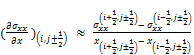(6)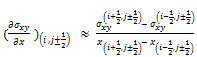(7)

2.   Interpolate spatial derivative in x-direction of the stress components cxx and σxy from positions (i,j±1/2)to position (i,j):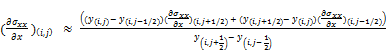(8)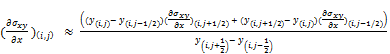(9)

3.   Approximate the spatial derivatives in y-direction of the stress components σyy and σxy at positions (i±1/2,j) using the finite difference quotient: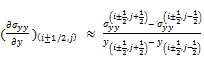(10)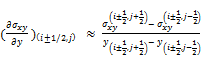(11)

4.   Interpolate spatial derivatives in y-direction of the stress components σyy and σxy from positions (i±1/2,j) to position (i, j):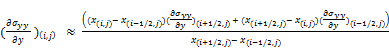(12)(13)

5.   Multiply the spatial derivatives of the stress components with 1/ρ that is defined at position(i,j):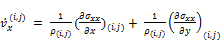(14)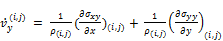(15)

For getting the three equations for stress (Equations 3 - 5) at the centre position (i-1/2, j-1/2), a similar procedure has to be performed.

1.   Approximate the spatial derivatives in x-direction of the velocity components vx and vy at positions (i-1/2,j-1/2±1/2) using a finite difference quotient: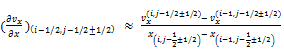(16)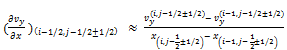(17)

2.   Interpolate spatial derivative in x-direction of the velocity components vx and vy from positions (i-1/2,j-1/2±1/2)to position (i-1/2,j-1/2):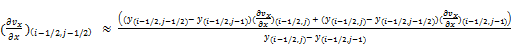(18)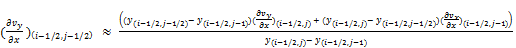(19)

3.   Approximate the spatial derivatives in y-direction of the velocity components vx and vy at positions (i-1/2±1/2,j-1/2) using a finite difference quotient: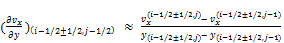(20)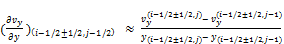(21)

4.   Interpolate spatial derivatives in y-direction of the velocity components vx and vy from positions (i-1/2±1/2,j-1/2)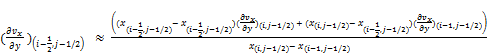(22)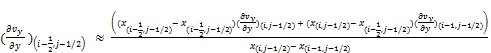(23)

5.   Multiply the spatial derivatives of the velocity components with the elastic moduli K and µ that are defined at position(i-1/2,j-1/2):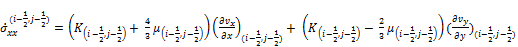(24)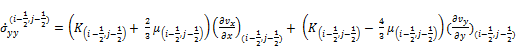(25)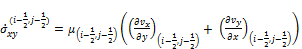(26)

From equation 24 - 26, it can be seen that the elastic moduli K and µ only need to be defined at the center position of one elementary finite difference cell. Elastic moduli do not occur at any other position in the grid. This is different to the widely - used fully staggered grid method (Virieux, 1986), where elastic moduli occur at different position within the one elementary cell. If material boundaries occur in the numerical domain, the numerical grid is built up in such a way that the approximated material boundaries run along boundaries between elementary cells. This way, the center positions of elementary cells always lie on either side of a material boundary and never on top of it. However, from equation 14 and 15, it can be seen that the density ρ needs to be defined at nodal points of the numerical grids. Nodal points can lie on top of material boundaries. For such nodal points, the density has to be arithmetically averaged from the materials of the four surrounding elementary cells.

The time derivatives in equation 14, 15, 24, 25 and 26 are approximated with an explicit finite difference approach: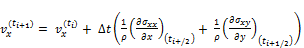(27)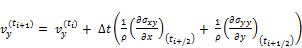(28)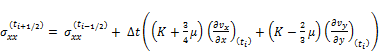(29)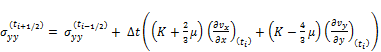(30)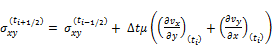(31)

In equation 27 - 31, the spatial indices i and j are removed compared to equations 14, 15, 25 and 26. The time index ti denotes any discrete time interval that is calculated in the numerical algorithm. Solutions for the stress components are calculated at half time increments. This procedure is the so called staggered time integration method (Virieux, 1986). Solutions of the velocity components (Equations 27 and 28) are already used for the solutions of the stress components. The time increment has to fulfill the von Neumann stability criterion that is calculated from the wave velocity and grid spacing of the numerical grid. (Higham., 1996; Seanger and Bohlen., 2004).

3. Results and Discussion

In this paper, to minimize the grid dispersion, the spatial sampling required at least 10 grid points per wavelength. Also, widely adopted Perfectly Matched Layer (PML) absorbing boundary (Collinos and Tsogka, 2001) is used and the source excitation which initializes the wave propagation is Gaussian which is implemented by adding a prescribed source time function to the source mesh, e. g, an explosion point source time function ( Ritcher wavelet, S(t)), such that:

τxx or zz(source grid) = τxx or zz (FD solution at source grid) + S(t) (32)

Unlike the conventional finite-difference method, which uses a fixed grid-size and time - step for the entire model region, spatially variable grid-size and time-step are used to achieve the optimal computational efficiency. In this ongoing research, variable grid-size and time-step changes are used to achieve both accuracy and efficiency in the simulations involving the use of the staggered - grid finite-difference schemes providing in addition, optimal computational savings.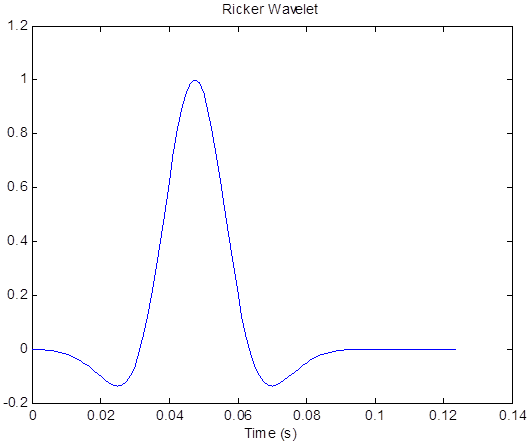Figure 2. Ricker wavelet set at frequency 20Hz.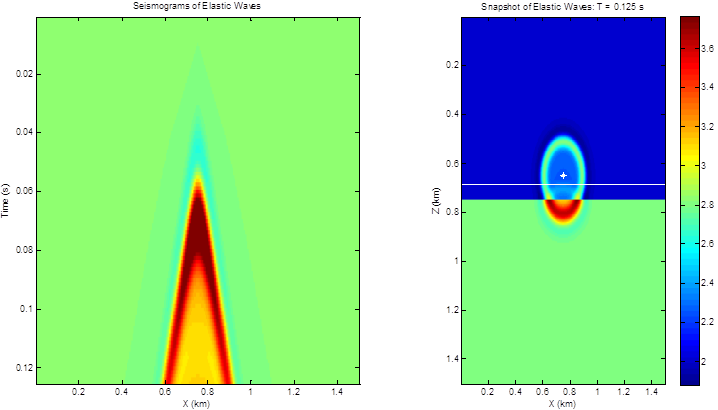Figure 3. Synthetic seismogram for the elastic wave propagated at input horizontal and vertical dimensions set at nx = nz = 300 (Time step nt =100, f =20Hz).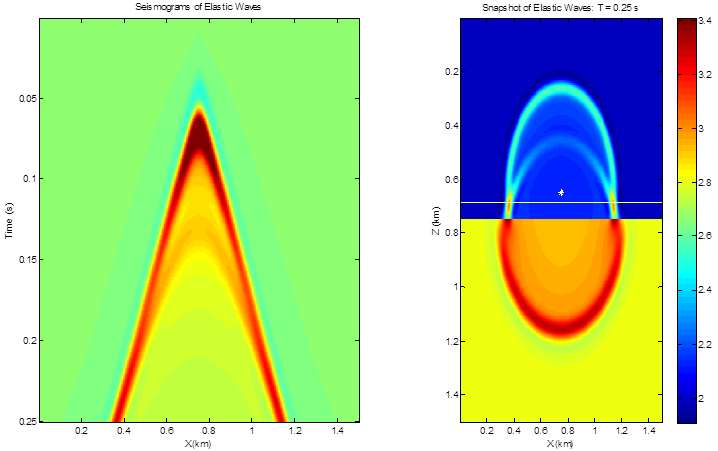Figure 4. Synthetic seismogram for the elastic wave propagated at input horizontal and vertical dimensions set at nx = nz = 300 (Time step nt = 200, f = 20Hz).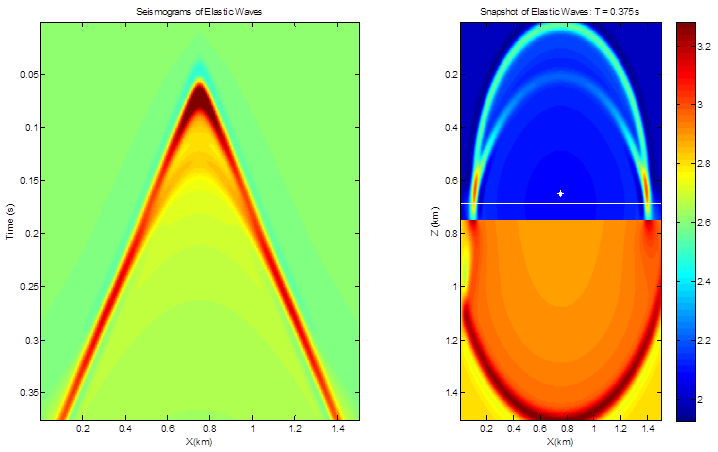Figure 5. Synthetic seismogram for the elastic wave propagated at input horizontal and vertical dimensions set at nx = nz = 300 (Time step nt =300, f =20Hz).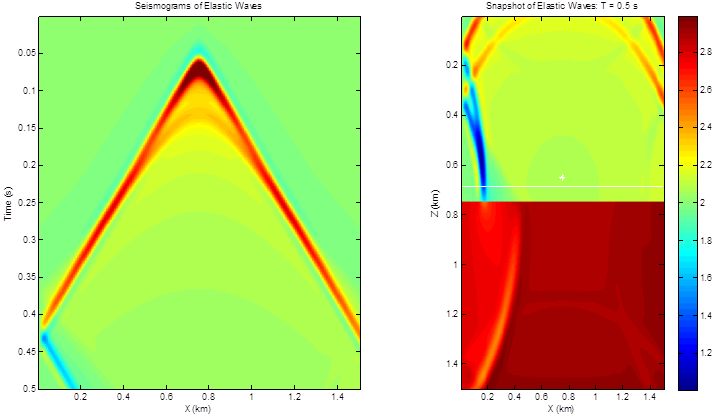Figure 6. Synthetic siesmogram for the elastic wave propagated at input horizontal and vertical dimensions set at nx = nz = 300 (Time step nt =400, f = 20Hz).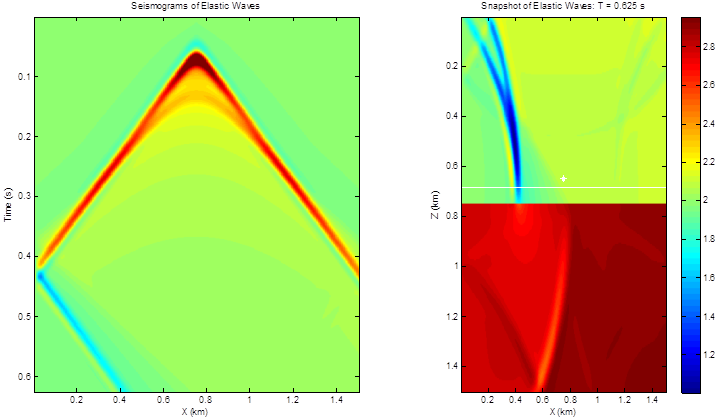Figure 7. Synthetic seismogram for the elastic wave propagated at input horizontal and vertical dimensions set at nx = nz = 300 (Time step nt =500, f = 20Hz).

For the figures shown in figures 3 - 7, a two layer model was used to model the speed of seismic waves propagating through a heterogeneous medium consisting of a low velocity layer overlying a high-velocity half space. The source is modeled using Ricker wavelength of frequency 20Hz (Figure 2). These figures shows a few snapshots of the seismic waves as they propagate away from the source at times 0.125s, 0.25s, 0.375s, 0.5s and 0.625s. Wavefronts from the source is propagated at a frequency of 20Hz. From 0 to 0.125s, the wave propagates majorly within the upper layer, after which the wave begins to interact with the boundary at approximately 0.75Km (750m). Upon interaction with the boundary, part of the wave is transmitted through the boundary, the refracted wave, and part bounces off the boundary, the reflected wave. From the snapshots shown in figure 6 and 7, as the refracted wave moves across the layer boundary, it generates a new wave type in the layer( head wave) that propagate upward to the surface.

Narrowing down on the effect of the grid-step sizes used for computing the synthetic seismogram for the wave propagated over an offset of 1500m with vertical and horizontal dimensions set at nx = nz = 300. The grid size used for the finite-difference is 5m x 5m x 5m having 260 x 260 x 100 grid points and the time step of 100, 200, 300, 400 and 500. The wavefield propagating is horizontally symmetric. The results of the simulations shows a clearer picture of the synthetic seismogram for time - stepping ranging from 400 - 500 as seen from figures 6 and 7 for a propagation time of 0.5s and 0.625s.

4. Conclusion

It is evident that the effect of grid step- sizes on computational time involved in the application of finite-difference scheme is crucial and needed to be properly handled to reduce numerically intensive complexity involve in seismic modeling as exemplify by the above model discussion which requires appropriate choice of having grid size 260 x 260 x 100 with data recorded for about 2s. This would be equivalent to about 1,000 time steps if done with 3D finite-difference scheme taking about 6 hours to regenerate a shot on parallel computers. In turn, this may require about 6,000 hours computing time using ordinary high configuration desktop computers with fairly large memory.

References

1. Bohlen, T. & E. H. Saenger., 2006: Accuracy of heterogeneous staggered-grid finite-difference modeling of Rayleigh waves, Geophysics, 71, No. 4, Pages T109-T115.
2. Collinos, F. and Tsogka, C., 2001, Application of the perfectly matched absorbing layer model to the linear elastodynamic problem in anisotropic heterogeneous media. Geophysics 66, No 1, 294 – 307.
3. Higham N. J., 1996. Accuracy and stability of numerical algorithms, Society for Industrial and applied Mathematics, Philadelphia, ISBN 0-8987 - 1355-2.
4. Kriiger, O. S., Saenger E. H., Oates, S. J and Shapiro, S. A., 2007, A numerical study on reflection coefficients of fractured media. Geophysics, 72, No. 3. D61 – D67.
5. Kurzmann, A. Przebindowska, A, Köhn, D. and Bohlen, T., 2013: Acoustic full waveform tomography in the presence of attenuation: a sensitivity analysis, Geophysical Journal International, 195 (2), 985 – 1000.
6. Moczo, P., Jozef, K and Halada, L.,(2004), The finite-difference method for seismologist.
7. Moczo, P., J. Kristek, M. Galis., P.Pazak., M.Balazovjech., 2007, The Finite-Difference and Finite-Element Modeling of Seismic Wave Propagation and Earthquake motion. Acta Physica Slovaca, 57, No. 2, 177-406.
8. Olowofela, J. A., Akinyemi, O. D., and Ajani, O. O.,(2015): Stability analysis for finite-difference schemeused for seismic imaging using amplitude and phase portrait. Applied and Computational Mathematics 4(1): 1-6.
9. Saenger, E. & T. Bohlen., 2004: Finite-difference modeling of viscoelastic and anisotropic wave propagation using the rotated staggered grid, Geophysics, 69, No. 2, 583-591.
10. Virieux, J., 1986, P – SV wave propagation in heterogeneous media: Velocity – Stress finite-difference method: Geophysics, 51, 889-901.

 Contents 1. 2. 3. 4.
Article ToolsAbstractPDF(503K)